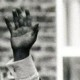# The beauty of the Riemann sphereReading Elliptic Tales: Curves, Counting, and Number Theorytoday, I came across the Riemann Sphere – a thing of beauty: a closed surface of infinite extent.

I will explain the maths of the sphere in a moment, but I am left wondering if one of two things apply:

(a) We are terrible at teaching maths in this country because although it produces such beautiful concepts too many children – perhaps a majority – think it is a thing of drudgery; or

(b) The appeal of maths is inherently limited and the number of people fascinated by a concept as seemingly contradictory as a closed surface of infinite extent is always going to be limited.

Anyway, here’s an explanation of the sphere…

We want to think about infinity but we will, at first, restrict our thinking to a single, straight line. We can say how far along this line we are by measuring the distance from an arbitrary starting point. So, we could be 1 unit along,$\pi$ units along or$-\pi$ units along and so on.

In fact we can represent where we are on the line by a ratio$\frac{a}{b}$ and represent each point in the form$a:b$. So$\pi$ can be$\pi:1$ for instance, so we are not restricted to the rationals in this form.

Now , think of this as one way to get further and further from our arbitrary starting point – first we go 1 unit and so are at$1:1$ then we go to$1:\frac{1}{2}$ then$\frac{1}{3}$ and so on – and we approach the limit$1:0$ which is the furthest we can possibly be and which we call infinitely far away from our starting point.

But what if we went in the other (negative) direction? Then we’d go to$1:-1$,$1:-\frac{1}{2}$ and so on … but at the limit we’d go to$1:0$ – exactly the same place we’d go to if we started in the opposite direction: hence the “negative” and “positive” infinities are the same and this line is actually a loop.

To go from this loop to the sphere we need to consider complex numbers. Here every number is of the form$a + b\Im$, where$\Im$ is the square root of -1.

Then we have to plot numbers on a plane, not a line and so every number has two co-ordinates, one of the form we discussed above in the form of the reals – eg$1:\frac{1}{7}$ and one along the imaginary axis of the form$\Im:\frac{1}{7}$ – we thus we get an infinite set of infinite closed loops – a sphere.